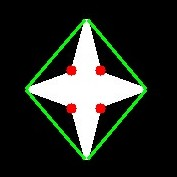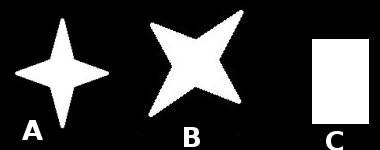OpenCV  3.1.0 Open Source Computer Vision
Contours : More Functions

## Goal

In this chapter, we will learn about

• Convexity defects and how to find them.
• Finding shortest distance from a point to a polygon
• Matching different shapes

## Theory and Code

### 1. Convexity Defects

We saw what is convex hull in second chapter about contours. Any deviation of the object from this hull can be considered as convexity defect.

OpenCV comes with a ready-made function to find this, cv2.convexityDefects(). A basic function call would look like below:

1 hull = cv2.convexHull(cnt,returnPoints = False)
2 defects = cv2.convexityDefects(cnt,hull)
Note
Remember we have to pass returnPoints = False while finding convex hull, in order to find convexity defects.

It returns an array where each row contains these values - **[ start point, end point, farthest point, approximate distance to farthest point ]**. We can visualize it using an image. We draw a line joining start point and end point, then draw a circle at the farthest point. Remember first three values returned are indices of cnt. So we have to bring those values from cnt.

1 import cv2
2 import numpy as np
3
5 img_gray = cv2.cvtColor(img,cv2.COLOR_BGR2GRAY)
6 ret, thresh = cv2.threshold(img_gray, 127, 255,0)
7 contours,hierarchy = cv2.findContours(thresh,2,1)
8 cnt = contours
9
10 hull = cv2.convexHull(cnt,returnPoints = False)
11 defects = cv2.convexityDefects(cnt,hull)
12
13 for i in range(defects.shape):
14  s,e,f,d = defects[i,0]
15  start = tuple(cnt[s])
16  end = tuple(cnt[e])
17  far = tuple(cnt[f])
18  cv2.line(img,start,end,[0,255,0],2)
19  cv2.circle(img,far,5,[0,0,255],-1)
20
21 cv2.imshow('img',img)
22 cv2.waitKey(0)
23 cv2.destroyAllWindows()

And see the result:image

### 2. Point Polygon Test

This function finds the shortest distance between a point in the image and a contour. It returns the distance which is negative when point is outside the contour, positive when point is inside and zero if point is on the contour.

For example, we can check the point (50,50) as follows:

1 dist = cv2.pointPolygonTest(cnt,(50,50),True)

In the function, third argument is measureDist. If it is True, it finds the signed distance. If False, it finds whether the point is inside or outside or on the contour (it returns +1, -1, 0 respectively).

Note
If you don't want to find the distance, make sure third argument is False, because, it is a time consuming process. So, making it False gives about 2-3X speedup.

### 3. Match Shapes

OpenCV comes with a function cv2.matchShapes() which enables us to compare two shapes, or two contours and returns a metric showing the similarity. The lower the result, the better match it is. It is calculated based on the hu-moment values. Different measurement methods are explained in the docs.

1 import cv2
2 import numpy as np
3
6
7 ret, thresh = cv2.threshold(img1, 127, 255,0)
8 ret, thresh2 = cv2.threshold(img2, 127, 255,0)
9 contours,hierarchy = cv2.findContours(thresh,2,1)
10 cnt1 = contours
11 contours,hierarchy = cv2.findContours(thresh2,2,1)
12 cnt2 = contours
13
14 ret = cv2.matchShapes(cnt1,cnt2,1,0.0)
15 print ret

I tried matching shapes with different shapes given below:image

I got following results:

• Matching Image A with itself = 0.0
• Matching Image A with Image B = 0.001946
• Matching Image A with Image C = 0.326911

See, even image rotation doesn't affect much on this comparison.